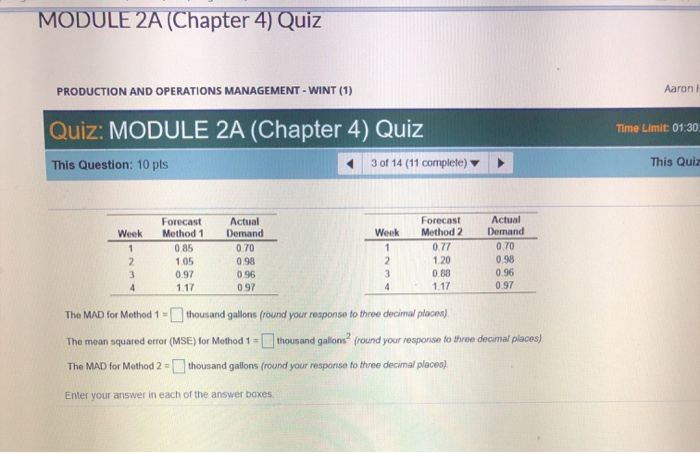# MODULE 2A (Chapter 4) Quiz Aaron H PRODUCTION AND OPERATIONS MANAGEMENT

Paper Format: APA

Pages: 1 Words: 275

Question

MODULE 2A (Chapter 4) Quiz Aaron H PRODUCTION AND OPERATIONS MANAGEMENT - WINT (1) Quiz: MODULE 2A (Chapter 4) Quiz Time Limit: 01:30 This Question: 10 pts This Quiz 3 of 14 (11 complete) Forecast Actual Forecast Method 1 Actual Demand Method 2 Demand Week Week 0.77 0.70 0.85 0.70 0.98 1.20 1.05 0.98 0 88 1.17 0.96 0.97 3. 0.96 0.97 1.17 0.97 The MAD for Method 1 =thousand gallons (round your response to three decimal places). %3D thousand gallons (round your response to three decimal places). The mean squared error (MSE) for Method 1 = thousand gallons (round your response to three decimal places). The MAD for Method 2 Enter your answer in each of the answer boxes.MAD is the average of absolute errors. Absolute error is the absolute value of the difference between actual and forecast values. MAD is determined by calculating the average value of the absolute errors.

MAD for method 1 = (ABS(0.70-0.85)+ABS(0.98-1.05)+ABS(0.96-0.97)+ABS(0.97-1.17))/4 (ABS stands for absolute value)

= 0.108 thousand gallons

MSE is determined by calculating the average of squared errors. Squared error is the square of the difference of actual and forecasted demand.

MSE for method 1 = ((0.70-0.85)^2+(0.98-1.05)^2+(0.96-0.97)^2+(0.97-1.17)^2)/4

= 0.017 thousand gallons2

MAD for method 2 = (ABS(0.70-0.77)+ABS(0.98-1.20)+ABS(0.96-0.88)+ABS(0.97-1.17))/4 (ABS stands for absolute value)

= 0.143 thousand gallons

MSE for method 2 = ((0.70-0.77)^2+(0.98-1.20)^2+(0.96-0.88)^2+(0.97-1.17)^2)/4

= 0.025 thousand gallons2